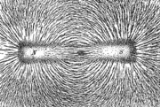xMagnetic fieldOverview

A magnetic field is a mathematical description of the magnetic influence of electric currents and magnetic materials. The magnetic field at any given point is specified by both a direction and a magnitude (or strength); as such it is a vector field
Vector field
In vector calculus, a vector field is an assignmentof a vector to each point in a subset of Euclidean space. A vector field in the plane for instance can be visualized as an arrow, with a given magnitude and direction, attached to each point in the plane...

.Technically, a magnetic field is a pseudo vector; pseudo-vectors, which also include torque and rotational velocity
Rotational speed
Rotational speed tells how many complete rotations there are per time unit. It is therefore a cyclic frequency, measured in hertz in the SI System...

, are similar to vectors except that they remain unchanged when the coordinates are inverted.
The magnetic field is most commonly defined in terms of the Lorentz force
Lorentz force
In physics, the Lorentz force is the force on a point charge due to electromagnetic fields. It is given by the following equation in terms of the electric and magnetic fields:...

it exerts on moving electric charges.